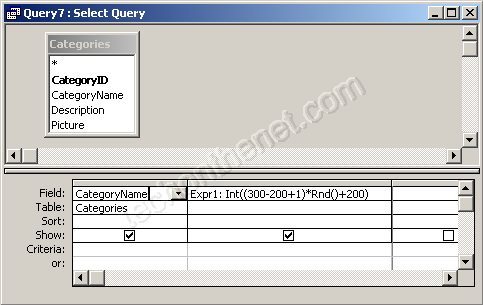## 8/05/2012

### Generate a random number between 2 user-specified values

In Access, the Rnd function allows you to generate a random number (integer value). You can specify the random number to be a value between 2 user-specified numbers.
The syntax for the Rnd function is:
Int ((upperbound - lowerbound + 1) * Rnd + lowerbound)
upperbound is the highest value that the random number can be.
lowerbound is the lowest value that the random number can be.

## For Example:

 Int ((6 - 1 + 1) * Rnd + 1) would return a random number between 1 and 6 Int ((200 - 150 + 1) * Rnd + 150) would return a random number between 150 and 200 Int ((999 - 100 + 1) * Rnd + 100) would return a random number between 100 and 999

## VBA Code:

The Rnd function can be used in VBA code. For example:
Dim LRandomNumber As Integer
LRandomNumber = Int ((300 - 200 + 1) * Rnd + 200)
In this example, the variable called LRandomNumber would now contain a random number between 200 and 300.

## SQL/Queries:

You can also use the Rnd function in a query.